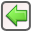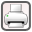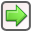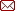﻿ Lighting Equipment Naming Convention > Lamp Naming Convention
 Navigation:  Lighting Equipment Naming Convention > Lamp Naming Conventionrev. 2008-01-10The lamp name is built up from:

technology + wattage + shape (where needed) + "v" + voltage (where not 120)

Technologies

 • i = incandescent, standard tungsten filament
 • q = quartz incandescent
 • f = fluorescent tube
 • mv = mercury vapour
 • hps = high pressure sodium
 • lps = low pressure sodium
 • mh = metal halide
 • n = neon
 • hq = halogen quartz
 • hir = halogen infra-red
 • cfl = compact fluorescent, external ballast not included
 • cfb = compact fluorescent with integral ballast on screw base
 • ht = halogen tungsten
 • led = light emitting diode

Shapes

 • t12 = 1.5" diameter tube
 • t8 = 1" diameter tube
 • ut12 = u-tube 1.5" diameter
 • ut8 = u-tube 1" diameter
 • par38 = parabolic reflectorized, 4.75" diameter
 • par30 = parabolic reflectorized, 3.75" diameter
 • par56 = parabolic reflectorized, 7" diameter
 • par20 = parabolic reflectorized, 2.5" diameter
 • a = standard residential bulb, slight pear shape
 • mr16 = low voltage compact halogen par, bi-pin base
 • g = globe
 • r40 = reflectorized 5" diameter
 • led = light emitting diode
 • dual = two compact fluorescent fingers
 • quad = four compact fluorescent fingers
 • globe = compact fluorescent enclosed to resemble an incandescent bulb
 • bx = long “biax” compact fluorescent lamp with 4-pin base